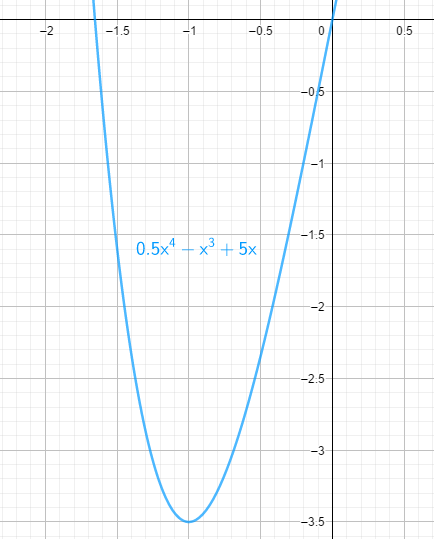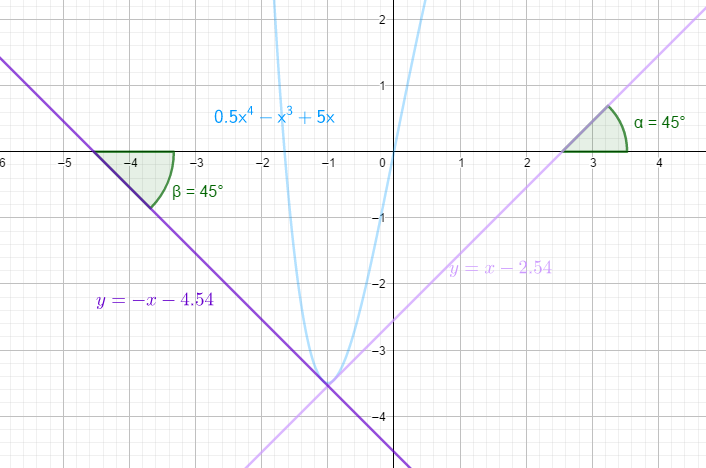### Math Notes

Subjects

#### Differential Calculus Solutions

##### Topics || Problems

Find the tangents to the curve $$y = \frac{1}{2} x^4 -x^3 +5x$$ which makes an angle of $$45^o$$ with the x-axis.$$y = \frac{1}{2} x^4 -x^3 +5x$$

$$\frac{dy}{dx} = 2x^3 -3x^2 +5$$

The slopes of the tangent lines is $$m = \tan 45 = 1$$ and $$m = \tan 135 = -1$$

Find the points of tangency along the curve.

$$\frac{dy}{dx} = 2x^3 -3x^2 +5 = 1$$

$$x_1 \approx -0.9108200822$$

$$x_2 \approx -1.0786168851$$

$$y$$ values

$$y_1 = \frac{1}{2} (-0.91)^4 -(-0.91)^3 +5(-0.91)$$

$$y_1 = -3.45437778711$$

$$y_2 = \frac{1}{2} (-1.08)^4 -(-1.08)^3 +5(-1.08)$$

$$y_2 = -3.46143951026$$

Thus the points of tangency are $$(-0.91, -3.45) ~ and ~ (-1.08, -3.46)$$

Find the first tangent.

$$y--3.45 = 1 (x--0.91)$$

$$y--3.45 = x+0.91$$

$$y = x -2.54$$

The second tangent.

$$y--3.46 =-1(x--1.08)$$

$$y--3.46 = -x-1.08$$

$$y = -x -4.54$$